# Division (mathematics) facts for kids

Kids Encyclopedia Facts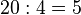$20: 4=5$

In mathematics, the word "division" means the operation which is the opposite of multiplication. The symbol for division can be a slash, a line, or the division sign (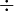$\div$), as in: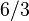$6/3\,$    or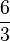$\frac 63$    or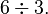$6 \div 3.$

Each, of those three, means "6 divided by 3" giving 2 as the answer. The first number is the dividend (6), and the second number is the divisor (3). The result (or answer) is the quotient. Whole numbers, any left-over amount is called the "remainder" (such as 14/4 gives 3 with the remainer as 2, as the number 324, same as 312 or 3.5).

The numbers can be very big, such as with two hundred: 200/5 = 40, or with 7 billion:  7,000,000,000 / 1000 = 7,000,000 (equals 7 million).

## With multiplication

If c times b equals a, written as: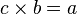$c \times b = a$

where b is not zero, then a divided by b equals c, written as: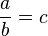$\frac ab = c$

For instance,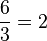$\frac 63 = 2$

since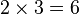$2 \times 3 = 6$.

In the above expression, a is called the dividend, b the divisor and c the quotient.

Division by zero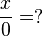$\frac x0 = ?$

...is not defined.

## Notation

Division is most often shown by placing the dividend over the divisor with a horizontal line, also called a vinculum, between them. For example, a divided by b is written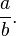$\frac ab.$

This can be read out loud as "a divided by b" or "a over b". A way to express division all on one line is to write the dividend, then a slash, then the divisor, like this: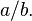$a/b.\,$

This is the usual way to specify division in most computer programming languages since it can easily be typed as a simple sequence of characters.

A typographical variation which is halfway between these two forms uses a slash but elevates the dividend, and lowers the divisor:

ab .

Any of these forms can be used to display a fraction. A fraction is a division expression where both dividend and divisor are integers (although typically called the numerator and denominator). A fraction is an accepted way of writing numbers. It is not always expected that the result of the division is written in decimals.

A less common way to show division is to use the obelus (or division sign) in this manner: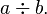$a \div b.$

But in elementary arithmetic this form is used rather often. The obelus is also used alone to represent the division operation itself, as for instance as a label on a key of a calculator.

In some non-English-speaking cultures, "a divided by b" is written a : b. However, in English usage the colon is restricted to expressing the related concept of ratios (with a:b said as "a is to b").

## Related pagesDivision (mathematics) Facts for Kids. Kiddle Encyclopedia.